Financial Analysis Techniques | IFT World
IFT Notes for Level I CFA® Program
IFT Notes for Level I CFA® Program

# Part 3

## 9. Liquidity Ratios

Liquidity ratios measure a company’s ability to meet short term obligations. It also indicates how quickly it turns assets into cash.

 Liquidity Ratios Current ratio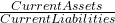Higher number implies greater liquidity. Quick ratio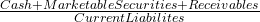Higher number implies greater liquidity. More conservative than current ratio as only more liquid current assets are included. Cash ratio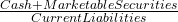This is the most conservative liquidity ratio, and a good measure of a company’s ability to handle a crisis situation. Defensive interval ratio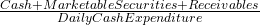Measures the number of days a company can operate before it runs out of cash. Higher number implies greater liquidity. Cash conversion cycle (net operating cycle) Days of inventory on hand (DOH) + Days of sales outstanding (DSO) – Number of days of payables The time between cash paid (to suppliers) and cash collected (from customers). A low number is better for the company as it means high liquidity. Long cash conversion cycle implies low liquidity

The example below for ABC Corp. illustrates the cash conversion cycle. The timeline for various events is illustrated below: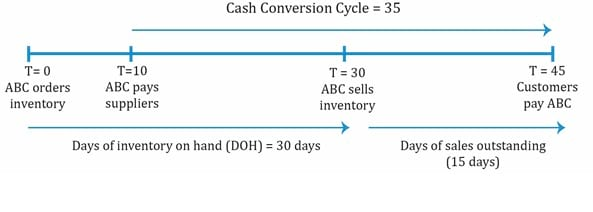## 10. Solvency Ratios

Solvency ratios measure a company’s ability to meet long term obligations. In simple terms, it provides information on how much debt the company has taken and if it is profitable enough to pay the interest on debt in the long term. It has to be analyzed within an industry’s perspective. Certain industries such as real estate use a higher level of leverage.

 Solvency Ratios Formula Interpretation Debt ratios Debt to assets ratio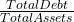Measures the amount of debt in total assets. Higher debt means low solvency and higher risk. A ratio of 0.5 implies 50% of assets are financed with debt. Debt to capital ratio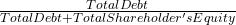Measures the amount of debt as a percentage of capital (debt + shareholder’s equity). Debt to equity ratio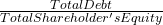Measures the amount of debt as a percentage of equity. Financial leverage ratio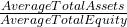Measures the amount of assets per unit of equity. Higher value means company is more leveraged. Coverage ratios Interest coverage ratio (also called ‘times interest earned’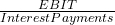Measures the company’s ability to make interest payments (how many times the company can make interest payments with its EBIT). Unlike the other solvency ratios, higher value for this ratio is better as it means stronger solvency. Fixed charge coverage ratio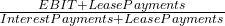Measures the ability of a company to pay interest on debt. Here, lease payments are added to EBIT as they are an obligation like interest payments. Like interest coverage ratio, higher value for this ratio implies stronger solvency.

Note that there are two categories of solvency ratios: debt (or leverage) ratios and coverage ratios.

In general, a high debt (or leverage) ratio implies a high level of debt, high risk and low solvency. With coverage ratios, a high number is good because this indicates high income relative to interest payments.

### 11. Profitability Ratios

 Profitability ratio Formula Interpretation Return on Sales Gross profit margin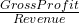Higher value means higher pricing and lower costs. Operating profit margin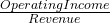Operating profit = gross profit – operating costs. Good sign if operating profit margin grows at a faster rate than gross profit margin. Pretax margin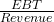EBT = operating profit – interest related expenses. Needs further analysis if pretax income increases only because of non-operating income. Net profit margin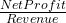Net profit = revenue – all expenses. Return on Investment Operating ROA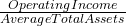For return, either net income or operating income (EBIT) can be used. Return on assets (ROA)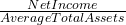For return, either net income or operating income (EBIT) can be used. Return on total capital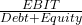Like operating ROA, EBIT is used. Measures return on capital before deducting interest. Return on equity (ROE)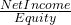A very important measure of return earned on equity capital. Unlike return on common equity, it includes minority and preferred equity. Return on common equity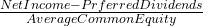Money available to common shareholders.

## 12 & 13. Integrated Financial Ratio Analysis

DuPont Analysis: The Decomposition of ROE

DuPont analysis decomposes a firm’s ROE to better analyze a firm’s performance.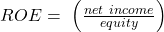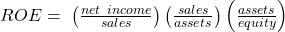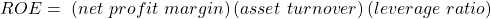Extended DuPont equation is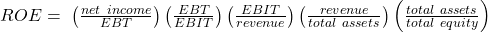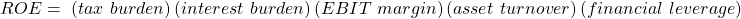Example

The following data is available for a company:

 2010 2011 2012 ROE 19% 20% 22% ROA 8.1% 8% 7.9% Total asset turnover 2 2 2.1

Based only on the information above, the most appropriate conclusion is that over the period 2010 to 2012, the company’s:

1. Net profit margin and financial leverage have decreased.
2. Net profit margin and financial leverage have increased.
3. Net profit margin has decreased but its financial leverage has increased.

Solution:

A quick glance at the data says profitability is going up and asset turnover has slightly increased from 2010 to 2012. ROA is going down from the second year.

First, break down ROE into: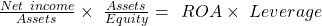ROE is going up (first row). Since ROA is going down, leverage must increase for ROE to increase. So A is incorrect.

Next, to determine if net profit margin increased or decreased, break down ROA into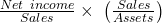. Since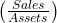or asset turnover is increasing, net profit margin has to decrease for return on assets to decrease. So, the correct answer is C.

Crash Courses for November CFA Level I, II exams are coming soon!
This is default text for notification bar